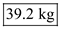## Quiz 6 : Batching and Other Flow InterruptionsLooking for Management Homework Help?# Quiz 6 : Batching and Other Flow Interruptions

The capacity of a system of process is determined by the capacity of its bottleneck resource. Therefore, the capacity can only be improved by improving the bottleneck's capacity. When it comes to setup requirement and batch production, increasing batch size in the bottleneck station is always advantageous from the point of view of capacity. However, if there is a setup requirement in a non-bottleneck station, reducing the batch size may be more advantageous because it will reduce the inventory level and lead time. Note the following information form the question in order to compute the capacity of the stamping machine and recommended batch size. Setup time to produce part A = 120 minutes Unit processing time for part A = 1 minute Setup time to produce part B = 120 minutes Unit processing time for part B = 0.5 minutes Batch size for the final assembly = 360 units a. Each final assembly consists of one unit of part A and two units of part B. So,Compute the capacity of stamping machine using the following formula:Compute the capacity in units per hour as follows:So, the capacity of the stamping machine is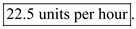b. Compute the flow rate of the assembly process as follows: There are 12 workers in the assembly process. Each final assembly takes 27 minutes to complete. Therefore, the effective flow rate is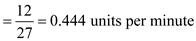Compute the recommended batch size using the following formula:So, the recommended batch size will be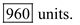The capacity of a system of process is determined by the capacity of its bottleneck resource. Therefore, the capacity can only be improved by improving the bottleneck's capacity. When it comes to setup requirement and batch production, increasing batch size in the bottleneck station is always advantageous from the point of view of capacity. However, if there is a setup requirement in a non-bottleneck station, reducing the batch size may be more advantageous because it will reduce the inventory level and lead time. Calculate the capacity of the process using the following data Setup (calibration) time for the urine test = 30 minutesPresent batch size for the urine test = 300 samples Setup (calibration) time for the blood test = 20 minutesProcessing time for complete blood test per sample = 1.5 minutes Present batch size for the blood test = 100 samples (70 basic and 30 complete tests) a. Compute the capacity using the following formula: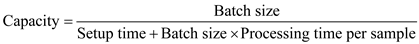So,So, the capacity is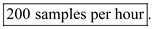b. The required capacity is 2.5 samples per minute.So, to attain the given capacity, the batch size should be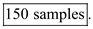c. Use the following formula to compute the capacity of basic blood test.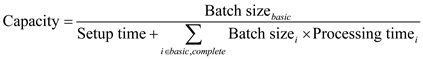So,So, the capacity of conducting the basic blood test is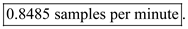The capacity of a system of process is determined by the capacity of its bottleneck resource. Therefore, the capacity can only be improved by improving the bottleneck's capacity. When it comes to setup requirement and batch production, increasing batch size in the bottleneck station is always advantageous from the point of view of capacity. However, if there is a setup requirement in a non-bottleneck station, reducing the batch size may be more advantageous because it will reduce the inventory level and lead time. a. Use the following formula to compute the recommended batch size to minimize the overall production/ inventory and still satisfying the demand as follows: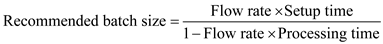The overall flow rate will be the total demand rate i.e.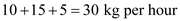. The overall setup time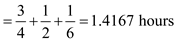The processing time for one kg is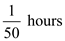So,Rounding off, the optimal batch size is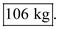b. The production of a particular item should be produced as per the demand ratio. So,So, the ideal batch size for the product F is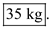c. Compute the maximum inventory using the following formula: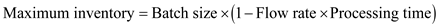The flow rate for the product C is 15 kg per hour. The processing time isSo,So, the maximum inventory of product C will be